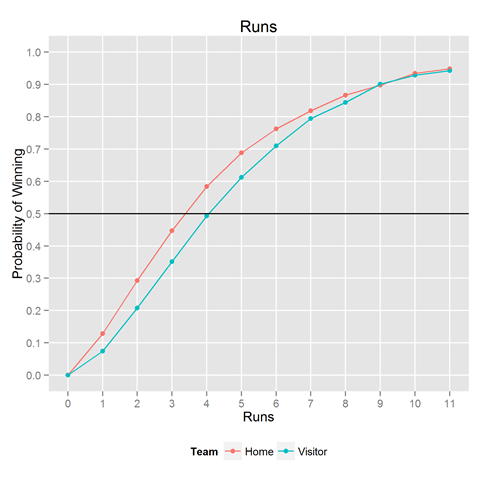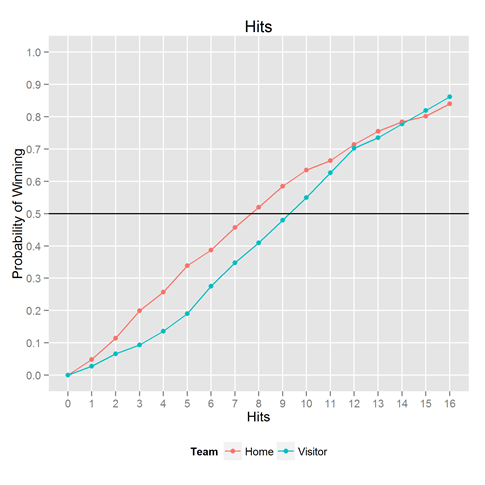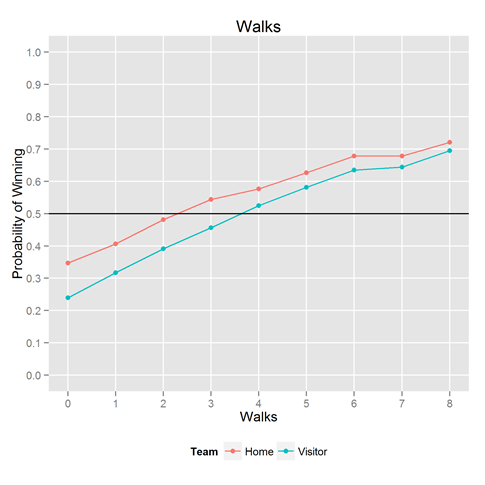Want to share your content on R-bloggers? click here if you have a blog, or here if you don't.

SIMPLY COUNTING OVER 44 YEARS OF DATAWe have a father-in-law who likes baseball. Occasionally, he asks us to figure out things, which we are more than happy to do. The last request was to figure out:

If a team scores X runs, what’s the probability it will win the game?

Luckily, we had the data to solve this problem (as mentioned in past posts). Looking back over 44 years of baseball games, we looked at how often a home team scored 1 run, and counted how often the home team won. We then looked at 2, 3, 4 runs, up to 11 runs. We stop at 11 runs because we only wanted to compute relative frequencies when there’s a decent amount of data. In all our analyses here, we cut the x-axis when there are fewer than 500 observations per bin. We analyzed the visiting team’s scores separately, to see the effect of the home team advantage.

The result is shown above. If you consistently score 3-4 runs a game, you’re winning about half the games. It’s simply not good enough. Going from 2 runs a game to 6 runs a game means going from winning 25% of the time to winning 75% of the time–all the difference in the world.

Because we had the data handy, we couldn’t help but looking at the same thing for the other key statistics: hits, walks, and errors. Results below.Want to play with it yourself? The R / ggplot2 code that made this plot is below. ggplot and dplyr are Hadley Wickham creations.

` ### Read in Data ### #Data obtained from http://www.retrosheet.org/ #Go for the files http://www.retrosheet.org/gamelogs/gl1970_79.zip through #http://www.retrosheet.org/gamelogs/gl2010_13.zip and unzip each to directories #named "gl1970_79", "gl1980_89", etc, reachable from your working directory. library(dplyr) library(ggplot2) #Column headers, Can get from http://www.dangoldstein.com/flash/Rtutorial2/cnames.txt #If you want all the headers, create from www.dangoldstein.com/flash/Rtutorial2/glfields.txt LabelsForScript=read.csv("cnames.txt", header=TRUE) #Loop to get together all data dat=NULL for (baseyear in seq(1970,2000,by=10)) { endyear=baseyear+9 #string manupulate pathnames #reading in datafiles to one big dat goes here for (i in baseyear:endyear) { mypath=paste("gl",baseyear,"_",substr(as.character(endyear),start=3,stop=4),"/GL",i,".TXT",sep="") cat(mypath,"n") dat=rbind(dat,read.csv(mypath, col.names=LabelsForScript\$Name)) }} #Force feed in the last few years for(mypath in c("gl2010_13/GL2010.TXT", "gl2010_13/GL2011.TXT", "gl2010_13/GL2012.TXT", "gl2010_13/GL2013.TXT")) {cat(mypath,"n") dat=rbind(dat,read.csv(mypath, col.names=LabelsForScript\$Name))} rel=dat[,c("Date", "Home", "Visitor", "HomeLeague", "VisitorLeague", "HomeScore", "VisitorScore", "Hhits", "Vhits", "Hwalks", "Vwalks", "Herrors", "Verrors" )] #relevant set rel\$year=substr(rel\$Date,start=1,stop=4) #################### #dplyr awesomeness. so fast, so good. srel_smart =rel %>% filter(VisitorLeague=="NL" & HomeLeague=="NL") %>% mutate(HW = HomeScore>VisitorScore, VW = VisitorScore>HomeScore) srel_smart=with(srel_smart,data.frame( Runs=c(HomeScore,VisitorScore), Hits=c(Hhits,Vhits), Walks=c(Hwalks,Vwalks), Errors=c(Herrors,Verrors), outcome=c(HW,VW), Team=c(rep("Home",nrow(srel_smart)),rep("Visitor",nrow(srel_smart))) )) ##### #Now with Runs srel_runs = srel_smart %>% group_by(Runs,Team) %>% summarise(Probability_Winning=round(mean(outcome),4), obs=length(outcome)) LIM=12 #ggplot. so pretty. so good. p=ggplot(subset(srel_runs,Runs p=p+geom_point() p=p+geom_line() p=p+theme(legend.position="bottom",panel.grid.minor=element_blank()) p=p+scale_x_continuous(breaks=0:LIM) p=p+scale_y_continuous(limits=c(0,1),breaks=seq(0,1,.1)) p=p + labs(title = "Runs",x="Runs",y="Probability of Winning") p=p+geom_hline(yintercept=.5) p ggsave(plot=p,file="srel_runs.png",height=6,width=6) ### #Now with hits srel_hits = srel_smart %>% group_by(Hits,Team) %>% summarise(Probability_Winning=round(mean(outcome),4), obs=length(outcome)) LIM=17 p=ggplot(subset(srel_hits,Hits p=p+geom_point() p=p+geom_line() p=p+theme(legend.position="bottom",panel.grid.minor=element_blank()) p=p+scale_x_continuous(breaks=0:LIM) p=p+scale_y_continuous(limits=c(0,1),breaks=seq(0,1,.1)) p=p + labs(title = "Hits",x="Hits",y="Probability of Winning") p=p+geom_hline(yintercept=.5) p ggsave(plot=p,file="srel_hits.png",height=6,width=6) #### #Now with walks srel_walks = srel_smart %>% group_by(Walks,Team) %>% summarise(Probability_Winning=round(mean(outcome),4), obs=length(outcome)) LIM=9 p=ggplot(subset(srel_walks,Walks p=p+geom_point() p=p+geom_line() p=p+theme(legend.position="bottom",panel.grid.minor=element_blank()) p=p+scale_x_continuous(breaks=0:LIM) p=p+scale_y_continuous(limits=c(0,1),breaks=seq(0,1,.1)) p=p + labs(title = "Walks",x="Walks",y="Probability of Winning") p=p+geom_hline(yintercept=.5) p ggsave(plot=p,file="srel_walks.png",height=6,width=6) #### #Now with errors srel_errors = srel_smart %>% group_by(Errors,Team) %>% summarise(Probability_Winning=round(mean(outcome),4), obs=length(outcome)) LIM=4 p=ggplot(subset(srel_errors,Errors p=p+geom_point() p=p+geom_line() p=p+theme(legend.position="bottom",panel.grid.minor=element_blank()) p=p+scale_x_continuous(breaks=0:LIM) p=p+scale_y_continuous(limits=c(0,1),breaks=seq(0,1,.1)) p=p + labs(title = "Errors",x="Errors",y="Probability of Winning") p=p+geom_hline(yintercept=.5) p ggsave(plot=p,file="srel_errors.png",height=6,width=6) `

The post Baseball: Probability of winning conditional on runs, hits, walks and errors appeared first on Decision Science News.